## Sunday, 28 August 2011

### Structural equation modeling in OpenMx: RAM path method

Suppose we have collected data on two verbal tests, words(W) and syntax (S), and two nonverbal tests, blocks (B) and pics (P), in a sample of 80 people. We want to test the hypothesis that the verbal tests are indicators of an underlying verbal factor (V), and the nonverbal tests are indicators of an underlying nonverbal factor (N), but the two factors are entirely independent. Before proceeding, it should be noted that this is a small sample size for testing such a model, but we use it to make it easy for users to inspect the dataset.

The key features of this model are easy to capture in a diagram , as in Figure 4. As is traditional in structural equation modeling (SEM), observed variables are shown in squares, and latent (i.e. postulated explanatory) variables in circles (or ellipses). Single-headed arrows represent regression coefficients, and double-headed arrows represent variances or covariances.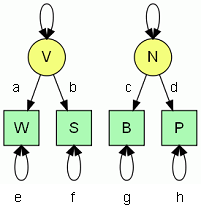Figure 4: Path diagram for 2 factor model

A structural equation model is principally concerned with explaining relationships between variables, rather than means. The information we have for testing our model comes from the covariance matrix between our four observed variables. Our model allows us to compute an expected covariance matrix, which can be compared with the observed matrix. Note that the diagonal of a covariance matrix contains the variances of the variables, which are equivalent to the covariance of a variable with itself.

A path diagram like Figure 4 can be converted into a set of structural equations that allow estimation of the size of the paths, given the observed covariance matrix. The expected covariance between any two observed variables can be estimated by applying path-tracing rules, which explain which paths should be multiplied and added to give a formula for the covariance. A full account of path-tracing rules can be found here.

1. Trace backward along an arrow and then forward, or simply forwards from one variable to the other but never forward and then back
2. Pass through each variable only once in each chain of paths
3. Trace through at most one two-way arrow in each chain of paths

For our simple model, this is really quite straightforward. The covariance between W and S is ab, because we trace back along path a, then forward (via V) along path b, and multiply the two paths. The covariance between W and B is zero, because there are no paths linking the two variables. The variance of W is a2 + e, where e is the portion of variance not accounted for by the model, i.e. the residual variance.
As an exercise, you should complete the following covariance matrix: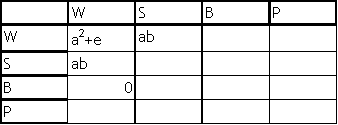The RAM path method allows one to compute expected covariances from a model using matrix algebra. It uses the path diagram specification to derive three matrices: S, A and F.
• A is for the asymmetric paths, or one-headed arrows.
• S is for the symmetric paths (two-headed arrows) and is symmetric.
• F is for filtering the observed variables out of the whole set (see below).
Let the number of observed variables be mobs, and number of latent variables by mlat, and mtot = mobs+mlat.
The dimensions of matrices A and S are both mtot × mtot,

For the model in Figure 4,

A =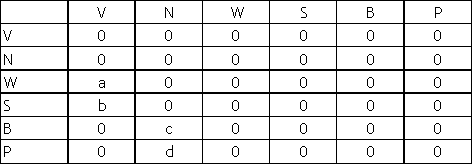S =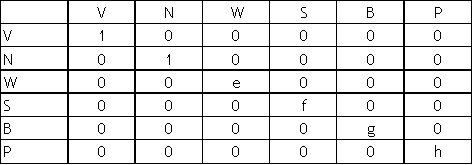Note that cell(1,1) and cell (2,2) of matrix S, which correspond to variances of the latent variables, are fixed to one. In effect, we standardize our latent variables, to avoid having too many parameters to estimate in the model.

F is a matrix of size mobs x mtot, and has a value of 1 for each cell where the row and column are the same.
F =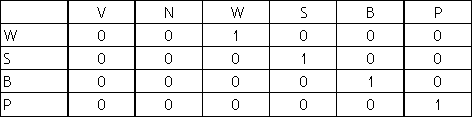Now that we have defined these matrices, plus the identiy matrix I (size mtot x mtot, with ones on diagonals and zeroes elsewhere), the expected covariance matrix can be computed by a matrix formula:

F * (I-A)-1 * S * ((I-A) -1)' * F'

We shall now demonstrate this with an example. Let us assume that the true values of the paths in Figure X are as follows: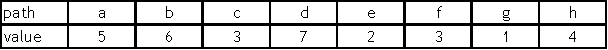You should be able to complete this table by hand, using the formulae derived above from path tracing rules
Expected covariance matrix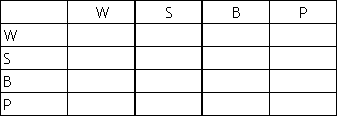Now, using the specifications given above, create matrices A, S and F.

You can do this most efficiently by creating a blank matrix, and then adding non-blank values using edit. To make a blank 6 x 6 matrix, type:
myA=matrix(c(0),nrow=6,ncol=6)
then type
myA= edit(myA)
and you can type in the nonblank values. Another way to do this is by specifying an element by its row and column index. So row 3, column 1 of the A matrix corresponds to a = 5, which can be achieved by
myA[3,1] = 5
Note that square brackets are used here.

Matrix S has values only on the diagonal, so we can take a short-cut and use the diag command to make a matrix with ones on all diagonals (an Identity matrix) and then edit to alter the non-one values:
myS=diag(1,6) # first index is the value, and second gives matrix size (square matrix)

Since the RAM path formula also requires an identity matrix, you could specify myI=myS, before modifying the myS matrix.

Once you have created matrices A, S, F, and I, you can plug them in to the RAM path formula,
expected covariance = F * (I-A)-1 * S * ((I-A) -1)' * F'

A good way of consolidating what you have learned so far is to see whether you can translate this formula into R code. Remember that the conventional way of representing matrix inversion is by -1, and that the ' symbol denotes a matrix transpose. Also remember that in R, matrix multiplication requires a sequence of symbols, not just *.

When you have worked out the formula, use it to compute myexpcov, and check that the values agree with those you computed by hand.

Degrees of freedom and model identification
An important feature of a structural model is that it should provide a parsimonious explanation of the data. We could simply use our optimization procedures to estimate the covariance matrix and means in our dataset, without having any model of their relationships, but this would be a theoretically vacuous exercise. As we shall see later on, we do in fact do such an estimation when evaluating a model, as it provides a baseline (a 'saturated model') against which to evaluate the likelihood of a structural model. But a good structural model will explain the data using fewer estimated parameters than observed statistics. The difference between the number of estimated parameters and observed statistics corresponds to the degrees of freedom of the model. For the model in Figure 4, assuming we set the variance of the latent variables to 1 and so do not have to estimate these values, we have a total of eight estimated parameters in the model; i.e. a, b, c, d, e, f, g and h. The observed covariance matrix that we input to the model contains 10 distinct values (remember that the symmetry of the matrix means that we do not need to count the covariances in the upper triangle, as they are the same as those in the lower triangle). This gives 10-8 = 2 degree of freedom(DF) In practice, in OpenMx, means as well as covariances are estimated, and for the model in Figure 4, this gives four additional observed values (giving total of 14) and four additional estimated parameters (giving total of 12), with the DF remaining at 2. It is important that a model has a positive DF: such a model is termed 'overidentified'. If you specify a model with more estimated parameters than observed variables it is underidentified and not amenable to sensible solution. As will be discussed further below, for some models, it may be necessary to give parameters a fixed value (so they do not count in the N estimated parameters), or to set two paths to the same value (so that two estimated parameters become one), in order to ensure the model is overidentified.

You are now ready to fit your model to a simulated dataset.
First let us simulate data, assuming that all variables have mean of 0, variance of 1, and that the covariance between W and S is .6, and between B and P is .5, with other covariances zero.
As a challenge, see if you can work out how to simulate a dataset with these characteristics using the mvrnorm command.

There are two methods in R for finding out more about how to use a command:
help(mvrnorm)
example(mvrnorm)

However, the help and examples in R are typically written with statisticians in mind, and are not always all that easy for others to understand.

It is possible that if you type these commands, you will just get an error message, i.e.
"No documentation for 'mvrnorm' in specified packages and libraries: you could try '??mvrnorm'"

Can you work out why this happens?

The answer is that this function is part of a package which is not automatically loaded when R starts up, unless you have saved a workspace image after loading it.
Check back to the first example script (Simcorr) and see what you need to do to fix this.

You should be able to work out how to use the mvrnorm command to create the whole set of data for 80 cases and 4 variables. You will need to input the correlation matrix for the 4 variables, and may find it helps if you write this down first. If you are stuck, you could modify the command in the "Generate correlated data" script to create one dataset for W and S (called 'myWS', with correlation of .6), and another for B and P (called 'myBP', with correlation .5). Since the two datasets are uncorrelated, this will work. You just need then to glue the two datasets together. The cbind command (for glueing matrix columns together) can be used for this purpose:
myscore=cbind(myWS,myBP)
(Note: there is an analogous command, rbind, for combining rows of matrices). N.B. if you do make your matrix this way, you may get slightly different results when you run the example script below, because the random numbers will be different from those you will get if you use mvrnorm to generate the full matrix in one step.

Check the correlation matrix for myscore, using cor(myscore). Note that the actual values for a sample of this size may deviate from those we specified; the expectation is for ones on the diagonals, zeros for correlations between verbal and nonverbal tests, .6 for correlations between verbal tests, and .5 for correlations between nonverbal tests. Use write.table to save 'myscore' with filename 'my4var'. See the "Simcorr" script if you can't remember how to do this.

We are now ready to use OpenMx to see how well our simulated data fit the model. OpenMx gives us an option of selecting model type 'RAM'. This is useful for beginners, because the model clearly maps on to the pictorial model in Figure 4. For a RAM-type model, we specify within the model the manifest variables, the latent variables, and the one-headed and two-headed paths between them, as in the example script below.
# -----------------------------------------------------------------------

# Program: PathCov_2factor, based on TwoFactorModel_PathCov.R

# Author: DVM Bishop

# Date: 4th March 2010

#

# Path style model input - Covariance matrix data input

# -----------------------------------------------------------------------

require(OpenMx)

#Prepare Data

myscore=read.table("my4var") # use the 'myscore' file that you created previously.

mylabels=c('W', 'S', 'B', 'P') # Put labels for the variables in a vector

colnames(myscore)=mylabels # Allocate the labels to mydata columns (our created dataset)

myFADataCov=cov(myscore) # Table of covariances will be compared with expected covs

# -----------------------------------------------------------------------

#Create an MxModel object; this won't execute until we do mxRun

# By specifying the type argument to equal ‘RAM’, we create a path style model

# -----------------------------------------------------------------------

db_twoFactorModel <- mxModel("DB_Two Factor Model_Path", type="RAM",

# type 'RAM' indicates we specify a RAM model for covariances

# and will use the mxPath function

mxData(

type="cov",

numObs=nrow(myscore),

),

manifestVars=c("W", "S", "B", "P"),

latentVars=c("V","N"),

# residual variances

mxPath(

from=c("W", "S", "B", "P"),

arrows=2, # 2-headed arrow for residual variance

free=TRUE, # All variances to be estimated

values=1, # Start value is 1

labels=c("e1","e2","e3","e4")

),

# latent variances ; NB no covariance between factors in this model

mxPath(

from=c("V","N"),

arrows=2, # 2-headed arrow for residual variance

free=FALSE, # if we wanted to allow for covariance between latent factors,

# would need to add ALL=true, to include 2-headed path between V and N

values=1, # Start value is 1

labels=c("varV","varN")

),

mxPath(

from="V",

to=c("W","S"),

arrows=1,

free=TRUE, # Can use single statement if you want to set all paths to free=TRUE

values=c(1,1), # Starting values

labels=c("a","b")

),

mxPath(

from="N",

to=c("B", "P"),

arrows=1,

free=c(TRUE,TRUE), # Just to illustrate alternative way of denoting true or false for each individual path

values=c(1,1),

labels=c("c","d")

)

) # end model definition

db_twoFactorFit <- mxRun(db_twoFactorModel)

summary(db_twoFactorFit)

db_twoFactorFit@output\$estimate

omxGraphviz(db_twoFactorModel, dotFilename = "twofact.dot")

# creates script for path diagram for the model and saves in format that can be read by Graphviz

#----------------------------------------------------------------

A RAM style model must include a vector of manifest variables (manifestVars=) and a vector
for latent variables (latentVars=). In this case the manifest variables are "W", "S", "B", and "P" and the latent variables are "V" and "N".
Paths are created using the mxPath function. Multiple paths can be created with a single invocation of the mxPath function. The 'from' argument specifies the path sources, and the' to' argument specifies the path destinations (also referred to as 'sinks'). If the ' to' argument is missing, then it is assumed to be identical to the ‘from’ argument. By default, the ith element of the ‘from’ argument is matched with the ith element of the ‘to’ argument, in order to create a path.

The arrows argument specifies whether the path is unidirectional (single-headed arrow, 1) or bidirectional (double-headed arrow, 2).
The next three arguments are vectors:
free takes a value of True or False, and specifies whether a path is free or fixed;
values is a numeric vector that specifies the starting value of the path;
labels is a character vector that assigns a label to each free or fixed parameter.

The summary function is a convenient method for displaying the highlights of a model after it has been executed. You should always check this table to ensure it is sensible.
For our model, the summary first gives a table of the distributional statistics of the data that was specified as 'observed=' in the mxData specification. If we use the 'raw' data option, then the statistics would show the means, etc, for the variables in the model. However, we specified a covariance matrix as observed data, and the summary will therefore give us means, etc. based for the covariances in the data matrix, which are less likely to be of interest. However, we have an opportunity to inspect means later on.
The next table shows the estimated values for parameters from matrix A (single-headed arrows), S (double-headed arrows), and M (means). Each path estimate has an associated standard error. This can be used to test whether the path differs significantly from zero. The 95% confidence interval around the path is (P-1.96s) to (P+1.96s), where P is the path coefficient, and s is the associated standard error. If this interval spans zero, the path is not significantly different from zero.
Thus for the first path, a, the 95% CI is:
.5835 – (1.96 * .21954) to .5835 + (1.96 * .21954)
i.e. from 0.153 to 1.014
Since this interval does not include zero, we can conclude that the path is significant and could not be dropped from the model without loss of fit.
The degrees of freedom is then given: you should always check this to be sure that you specified the model correctly and that the number of observed statistics and estimated parameters is as expected, and that the DF is positive.
The negative log likelihood of the model is multiplied by 2 (giving -2LL). You should be able to explain what this number represents: it is the lowest likelihood that was obtained by the optimization procedure when estimating model parameters. In estimating this value, we arrive at useful estimates of parameters, but the actual -2LL value is not meaningful on its own. It can, however, be compared with likelihoods of other models, to give an idea of whether the model provides a good account of the data.
There are two types of comparison that are useful. The first, which is automatically provided in OpenMx, is a comparison with the 'saturated model'. Although this is termed a 'model', it is not one in the normally accepted sense, since there are no latent variables in it. It simply represents the case where the optimization procedure is used to estimate the observed statistics. This is the kind of exercise we did with the two scripts showing likelihood calculations, first for a pair of variables, and then for three variables. The value of -2LL for a saturated model will always be lower than that for a model with latent variables, because the search for a fit is totally unconstrained. As noted above, however, the saturated model is of no theoretical interest, and it has an equal number of observed statistics and estimated values, and hence no degrees of freedom. The saturated model is of use, though, to provide a baseline level of -2LL against which to compare our overidentified model. If we subtract the -2LL value of the saturated model from the -2LL value for our model of interest, we get a statistic that follows the chi square distribution. The degree of freedom correspond to the DF for the model of interest minus the DF for the saturated model (which is zero). So, in this case:
chi square = 204.3614 – 201.7717 = 2.5897
DF = 2 – 0 = 2
As shown in the script output, the p-value corresponding to this chi square is .273, which is not significant. When fitting a structural model, a nonsignificant chi square is an indication of good model fit, because it means that the -2LL associated with the model is no different from the -2LL of the saturated model, even though our new model is more parsimonous.
The values of AIC, BIC and RMSEA are alternative methods for measuring goodness of fit of a model.

For our model, Akaike's Information Criterion (AIC) is less than zero, also indicating good fit. BIC refers to the Bayesian information criterion (BIC), where again, smaller is better. Finally, a usual rule of thumb is that the RMSEA statistic should be .05 or less for very good fit, or between .05 and .10 for good fit.

The last line of code allows you to export the path diagram corresponding to model as a .dot file. If you try to open this in a text browser, you will see a list of drawing commands. To convert these to a diagram, you need to download the free software Graphviz from:
Once downloaded, start up the Gvedit software, and Open the .dot file you created, (option from File menu). The Graphviz menu item then allows you to Run, which creates the path diagram, using .gif format as default. The path diagram is the same as that shown above, except that is also includes a triangle called 'one', with paths to all manifest variables. Triangles are used in structural equation models to represent constants: here the triangle is included because the means are also modeled in OpenMx. If you want to see the diagram without the means triangle, you can just delete all the statements referring to 'one' in the .dot file, and then re-run Gvedit.
As an exercise, you should now re-run the OpenMx script, but with a much larger dataset. Use the same method as before to generate a simulated dataset, with the same correlations between variables, but with 5000 cases. Save it under a new name. Re-run the script: you won't need to change anything other than the name of the dataset that is the argument of the 'read.table' command. Compare the output with that of the same model with the smaller dataset. How does the change in sample size affect the path estimates, the standard errors, the degree of freedom, and the measures of model fit?
Now repeat the exercise, but this time, have the paths transposed, so that W and B are indicators of V, and S and P are indicators of N. You can do this by simply modifying the mxPath statements for 'from = "V"' and 'from = "N"' so that the 'to' paths refer to "W" and "B" in the first case, and to "S" and "P" in the second case. The model specified in the script now gives a very poor fit to the data, because the pattern of correlations cannot be explained by the path structure, which implies correlations only between W and S, and B and P.
In a final test, return to the original script, reading in the data from 50 simulated cases. We are now going to see what happens if we drop one path. Usually we compare a model with the saturated model to test goodness of fit, but different models can be directly compared with one another if they are nested. Nested models are ones which have the same path structure, but which differ purely in terms of whether specific paths are fixed. If we set the starting value of a path to zero, and specify it as free=FALSE, this means that OpenMx will not estimate its value, but will keep it at the starting value: in effect this means the path is dropped. We will illustrate this by dropping path d. Before reading on, inspect the script and see if you can work out how to achieve this.
The answer is to change two lines of the following portion of script:
mxPath(
from="N",
to=c("B", "P"),
arrows=1,
free=c(TRUE,FALSE),
values=c(1,0),
labels=c("c","d")
The model now has one more degreeof freedom, and we can test the effect of dropping the path by computing chi square as follows: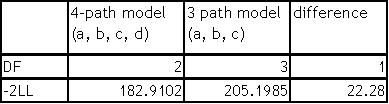A chi square of 22.28 with 1 DF has associated probability < .001. This tells us that dropping path d leads to highly significant worsening of fit of the model.
R will compute the p-value associated with a given value of chi square, by the expression:
1-pchisq(chi,df)
so, for this example, the probability can be computed as:
1-pchisq(22.28,1)
For readers who are used to using chi square to test for associations between variables, it may seem odd that a nonsignificant chi square is indicative of a good model fit, whereas a highly significant chi square denotes poor model fit. The key point to note is that in traditional statistical tests, chi square is compared against chance association, and we typically are interested in results that cannot be explained by chance. In contrast, in SEM the fit is tested against either the saturated model, or (as in this case) an overidentified model with fewer degrees of freedom. The further the estimated parameters are from the comparison model, the higher the chi square, and hence the more likely to be 'significant'. Significance here denotes a departure of estimated values from obtained values. Your experimentation above with varying sample size should also have illustrated a further point about model fit, which is that the larger the sample size, the more precise will be the estimated statistics (i.e. the smaller the standard errors), but also the more likely it is that a structural model will differ significantly from the saturated model.
You will find that this kind of experimentation with scripts using different datasets and manipulating the paths gives you a far better understanding of how SEM works than any amount of reading.

1.Thanks for this really essential, excellent tutorial. I'm working through it now, and hope to use it with fMRI time-course data...

Anyway, I've found I've got a bit stuck on getting the PathCov_2factor script working. I've generated the data with the following script:

#set.seed(0)
sigma = diag(c(1,1,1,1))
sigma[1,2] = 0.6;
sigma[2,1] = 0.6;
sigma[3,4] = 0.5;
sigma[4,3] = 0.5;
sigma
mydata = mvrnorm(80,c(0,0,0,0),sigma)
write.table(mydata,'~/sem_openmx/my4var')

I initially wrote out your script by hand, but have now copied and pasted the whole script into R. Either way, I find I have problems with the estimated values of the Std. Error which all appear as NaN. With much larger sample sizes (e.g. 8000) some of these become numbers, but are large (in comparison to the estimates).

Other parameters (such as the -2LL, etc) all seem reasonable:

free parameters:
name matrix row col Estimate Std.Error lbound ubound
1 a A W V 0.7692258 NaN
2 b A S V 0.8187957 NaN
3 c A B N 0.5022223 NaN
4 d A P N 0.5136759 NaN
5 e1 S W W 0.2756410 NaN
6 e2 S S S 0.3729581 NaN
7 e3 S B B 0.3859098 NaN
8 e4 S P P 0.6766048 NaN

observed statistics: 10
estimated parameters: 8
degrees of freedom: 2
-2 log likelihood: 212.9178
saturated -2 log likelihood: 209.5215
number of observations: 80
chi-square: 3.396338
p: 0.1830183

Any idea what might be the problem?

Thanks again for the blog, it's really helped :)

Mike [University of Edinburgh]

2.This comment has been removed by the author.

3.So this is caused by the inverted Hessian matrix (which is the covariance matrix) having negative values on the diagonal.

This is generally due to something being underdefined in the design? Is it a problem that the strength of the correlation between W and S can be modified by EITHER changing a or changing b? In some ways the model isn't defined well enough?

In the tutorial you show that there are 10 observed values (in the covariance matrix) and 8 unknown parameters, suggesting 2 dof. I'm a bit worried that the 0s in the covariance matrix don't help much - if that makes sense?...

Thinking about this a bit more: Given that this model can be split into two smaller models, surely these should be possible to estimate too? But when I count up the DoF for just the V,W,S sub-graph...

(the model looks like: V-(a)->W, V-(b)->S, V-(1)->V, W-(e)->W, S-(f)->S)

This gives us 4 unknowns (a,b,e,f), and only three values in the covariance matrix (a^2+e, b^2+f and ab)...

...doesn't this mean the model is "secretly" underdefined?

Just trying to figure this all out.

Hope my question makes sense!

-- Mike (lionfishy at gmail dot com).

4.Sorry for the delay in replying. As I mentioned at the start of this blog, I'm not an expert in either structural equation modeling or OpenMx, and the problem you describe foxes me too.
I did what I usually do, and Googled "'standard errors' NaN OpenMx", which ultimately led me to some discussion of the NaN standard error problem on the OpenMx site. It wasn't terribly illuminating, but the noninvertible Hessian was mentioned here:
5.